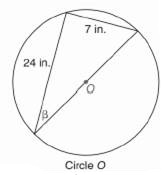Chapter 11.CR, Problem 8CR### Elementary Geometry for College St...

6th Edition
Daniel C. Alexander + 1 other
ISBN: 9781285195698

#### Solutions

Chapter
Section### Elementary Geometry for College St...

6th Edition
Daniel C. Alexander + 1 other
ISBN: 9781285195698
Textbook Problem
1 views

# In Exercises 5 to 8, state the ratio needed, and use it to find the measure of the indicated angle to the nearest degree.To determine

To find:

The measure of the angle indicated to the nearest degree.

Explanation

Procedure used:

In any right triangle ABC with mA=θ we have the following ratios.

sinθ=OppositeHypotenuse

Calculation:

Given:

The triangle inside the circle is a right triangle since, the diameter subtends right angle at its circumference.

Thus in ΔABC

AB=24 in.,

BC=7 in

### Still sussing out bartleby?

Check out a sample textbook solution.

See a sample solution

#### The Solution to Your Study Problems

Bartleby provides explanations to thousands of textbook problems written by our experts, many with advanced degrees!

Get Started

#### Solve the equations in Exercises 126. 1x211+1=0

Finite Mathematics and Applied Calculus (MindTap Course List)

#### Divide: 16x24x42x2

Elementary Technical Mathematics

#### True or False: converges.

Study Guide for Stewart's Single Variable Calculus: Early Transcendentals, 8th

#### The length of a = 4i – j – 2k is: 11 21

Study Guide for Stewart's Multivariable Calculus, 8th

#### Define content analysis and archival research.

Research Methods for the Behavioral Sciences (MindTap Course List)